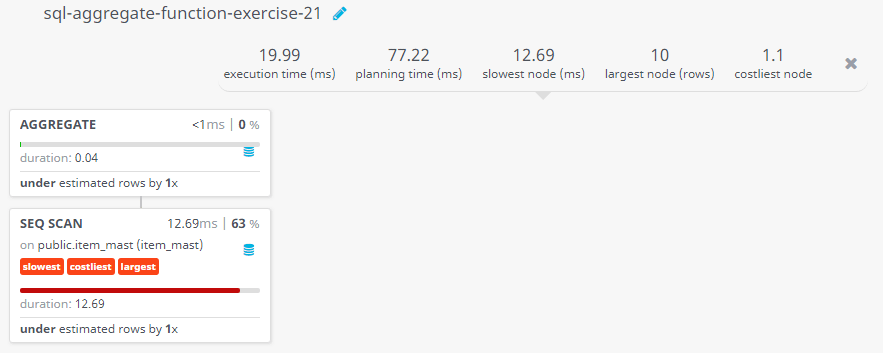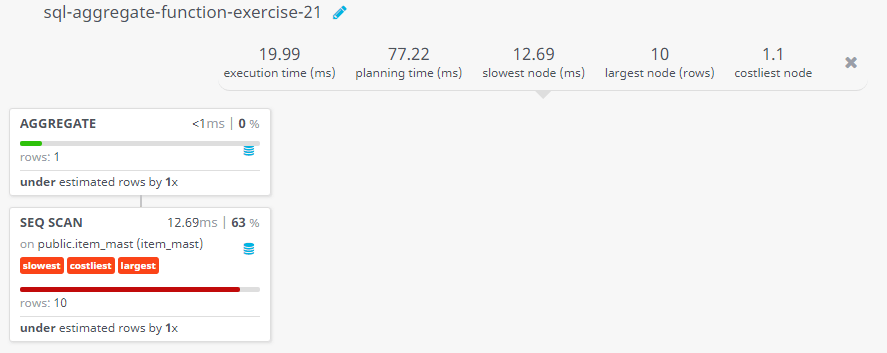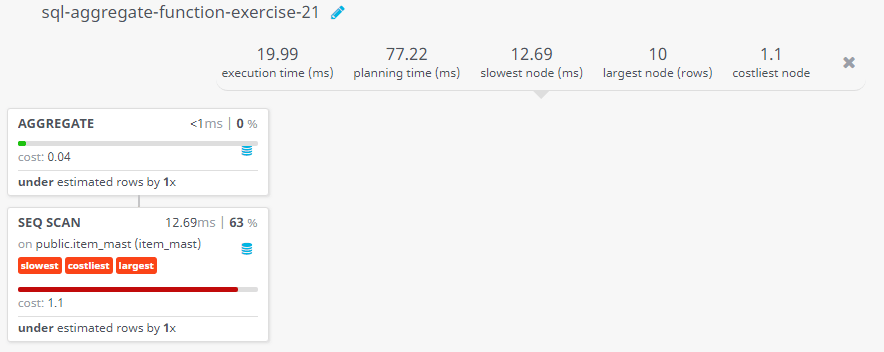﻿ SQL: Calculate the average price of all the products

# SQL Exercises: Calculate the average price of all the products

## SQL Aggregate Functions: Exercise-21 with Solution

From the following table, write a SQL query to calculate the average product price. Return average product price.

Sample table: item_mast

Sample Solution:

``````SELECT AVG(pro_price) AS "Average Price"
FROM item_mast;
``````

Output of the Query:

```Average Price
1435.0000000000000000
```

Code Explanation:

The said SQL query calculates the average price of all items in the 'item_mast' table. The "AVG(pro_price)" function calculates the average value of the "pro_price" column, and the "AS 'Average Price'" clause gives the result column a label of "Average Price".

## Query Visualization:

Duration:Rows:Cost:Have another way to solve this solution? Contribute your code (and comments) through Disqus.

Previous SQL Exercise: Count how many salesmen each day register orders.
Next SQL Exercise: Find the number of products over or equal to Rs 350.

What is the difficulty level of this exercise?

Test your Programming skills with w3resource's quiz.

﻿

## SQL: Tips of the Day

What is the best way to paginate results in SQL Server?

```SELECT  *
FROM    ( SELECT    ROW_NUMBER() OVER ( ORDER BY OrderDate ) AS RowNum, *
FROM      Orders
WHERE     OrderDate >= '1980-01-01'
) AS RowConstrainedResult
WHERE   RowNum >= 1
AND RowNum < 20
ORDER BY RowNum
```

Database: SQL Server

Ref: https://bit.ly/3MGrNlk

We are closing our Disqus commenting system for some maintenanace issues. You may write to us at reach[at]yahoo[dot]com or visit us at Facebook